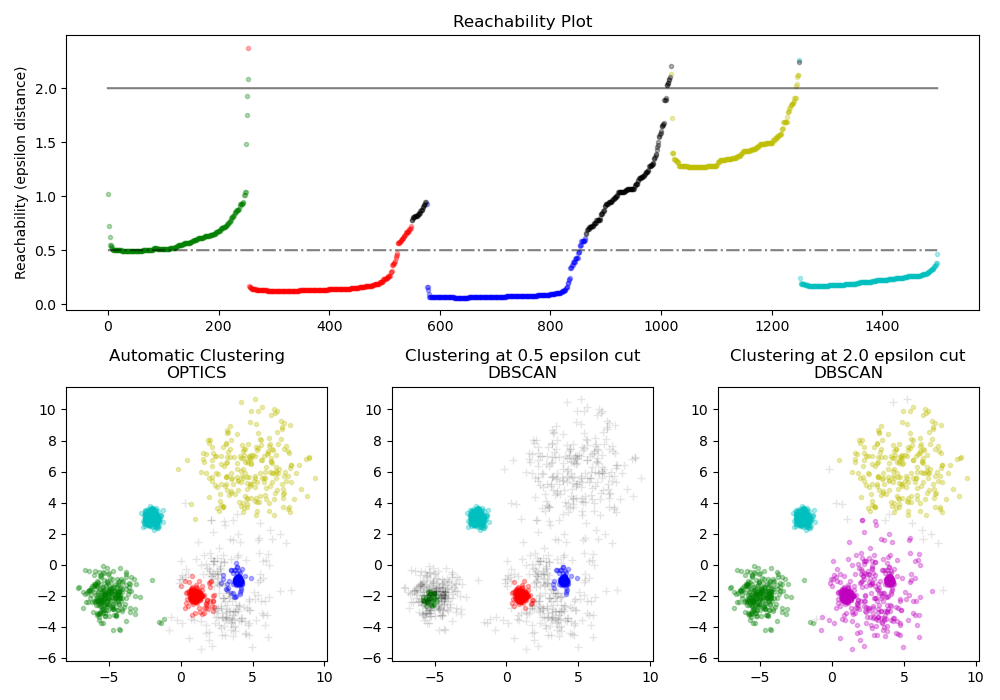# Demo of OPTICS clustering algorithm¶

Finds core samples of high density and expands clusters from them. This example uses data that is generated so that the clusters have different densities. The OPTICS is first used with its Xi cluster detection method, and then setting specific thresholds on the reachability, which corresponds to DBSCAN. We can see that the different clusters of OPTICS’s Xi method can be recovered with different choices of thresholds in DBSCAN.# Authors: Shane Grigsby <refuge@rocktalus.com>

from sklearn.cluster import OPTICS, cluster_optics_dbscan
import matplotlib.gridspec as gridspec
import matplotlib.pyplot as plt
import numpy as np

# Generate sample data

np.random.seed(0)
n_points_per_cluster = 250

C1 = [-5, -2] + 0.8 * np.random.randn(n_points_per_cluster, 2)
C2 = [4, -1] + 0.1 * np.random.randn(n_points_per_cluster, 2)
C3 = [1, -2] + 0.2 * np.random.randn(n_points_per_cluster, 2)
C4 = [-2, 3] + 0.3 * np.random.randn(n_points_per_cluster, 2)
C5 = [3, -2] + 1.6 * np.random.randn(n_points_per_cluster, 2)
C6 = [5, 6] + 2 * np.random.randn(n_points_per_cluster, 2)
X = np.vstack((C1, C2, C3, C4, C5, C6))

clust = OPTICS(min_samples=50, xi=0.05, min_cluster_size=0.05)

# Run the fit
clust.fit(X)

labels_050 = cluster_optics_dbscan(
reachability=clust.reachability_,
core_distances=clust.core_distances_,
ordering=clust.ordering_,
eps=0.5,
)
labels_200 = cluster_optics_dbscan(
reachability=clust.reachability_,
core_distances=clust.core_distances_,
ordering=clust.ordering_,
eps=2,
)

space = np.arange(len(X))
reachability = clust.reachability_[clust.ordering_]
labels = clust.labels_[clust.ordering_]

plt.figure(figsize=(10, 7))
G = gridspec.GridSpec(2, 3)
ax1 = plt.subplot(G[0, :])
ax2 = plt.subplot(G[1, 0])
ax3 = plt.subplot(G[1, 1])
ax4 = plt.subplot(G[1, 2])

# Reachability plot
colors = ["g.", "r.", "b.", "y.", "c."]
for klass, color in zip(range(0, 5), colors):
Xk = space[labels == klass]
Rk = reachability[labels == klass]
ax1.plot(Xk, Rk, color, alpha=0.3)
ax1.plot(space[labels == -1], reachability[labels == -1], "k.", alpha=0.3)
ax1.plot(space, np.full_like(space, 2.0, dtype=float), "k-", alpha=0.5)
ax1.plot(space, np.full_like(space, 0.5, dtype=float), "k-.", alpha=0.5)
ax1.set_ylabel("Reachability (epsilon distance)")
ax1.set_title("Reachability Plot")

# OPTICS
colors = ["g.", "r.", "b.", "y.", "c."]
for klass, color in zip(range(0, 5), colors):
Xk = X[clust.labels_ == klass]
ax2.plot(Xk[:, 0], Xk[:, 1], color, alpha=0.3)
ax2.plot(X[clust.labels_ == -1, 0], X[clust.labels_ == -1, 1], "k+", alpha=0.1)
ax2.set_title("Automatic Clustering\nOPTICS")

# DBSCAN at 0.5
colors = ["g", "greenyellow", "olive", "r", "b", "c"]
for klass, color in zip(range(0, 6), colors):
Xk = X[labels_050 == klass]
ax3.plot(Xk[:, 0], Xk[:, 1], color, alpha=0.3, marker=".")
ax3.plot(X[labels_050 == -1, 0], X[labels_050 == -1, 1], "k+", alpha=0.1)
ax3.set_title("Clustering at 0.5 epsilon cut\nDBSCAN")

# DBSCAN at 2.
colors = ["g.", "m.", "y.", "c."]
for klass, color in zip(range(0, 4), colors):
Xk = X[labels_200 == klass]
ax4.plot(Xk[:, 0], Xk[:, 1], color, alpha=0.3)
ax4.plot(X[labels_200 == -1, 0], X[labels_200 == -1, 1], "k+", alpha=0.1)
ax4.set_title("Clustering at 2.0 epsilon cut\nDBSCAN")

plt.tight_layout()
plt.show()


Total running time of the script: ( 0 minutes 1.061 seconds)

Gallery generated by Sphinx-Gallery